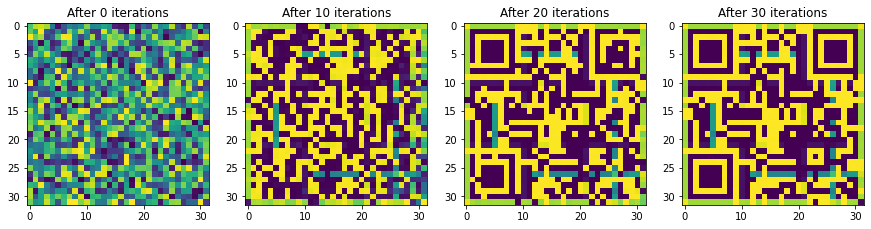This is my writeup for the only Misc challenge “Deaths Glance” in HTB University CTF 2022 (on CTFtime).

The challenge was initially labelled as “easy” at the beginning of the event, and was changed to “medium” after 2 hours into the CTF with no solves to this challenge. Our team was the 2nd solved and submitted flag to this challenge, about one or two hours after the challenge first been solved about 24 hours into the CTF. There were 6 solves to this challenge in the 54 hours CTF with 328 teams submitted at least one flag.

## The Challenge

The challenge is given with the following description:

You find yourself in possession of an ancient forbidden spell. Rumors have it that by revealing the rune originated from the spell, the mystery behind how you perish will be unveiled.

The challenge is also with a downloadable containing a `forbiden_spell.pt` file and a `challenge.py` file.

The Python file contains a convolutional neural network implemented in PyTorch, with the weights initialisation using a fixed seed, and code in comments defining some `dummy_data_size`. The model is for classification with 100 output classes, and input is 1-channel (greyscale) 32x32 image.

``````import torch
import torch.nn as nn
torch.manual_seed(50)
device = "cpu"

def weights_init(m):
if hasattr(m, "weight"):
m.weight.data.uniform_(-0.5, 0.5)
if hasattr(m, "bias"):
m.bias.data.uniform_(-0.5, 0.5)

class LeNet(nn.Module):
def __init__(self):
super(LeNet, self).__init__()
act = nn.Sigmoid
self.body = nn.Sequential(
act(),
act(),
act(),
act(),
)
self.fc = nn.Sequential(
nn.Linear(768, 100)
)

def forward(self, x):
out = self.body(x)
out = out.view(out.size(0), -1)
out = self.fc(out)
return out

net = LeNet().to(device)
net.apply(weights_init)
criterion = nn.CrossEntropyLoss()

"""
forbidden_spell = torch.load('path to challenge file')
dummy_data_size=torch.Size([1, 1, 32, 32])

#code here
"""
``````

Load and inspecting `forbidden_spell.pt`, it contains a list of torch tensors, the shape of the data in the file matches with the model parameters of the model defined in `challenge.py` . Their shape can be printed out with `for s in forbidden_spell: print(s.shape)` and `for parameters in net.parameters(): print(parameters.shape)` respectively, and gives:

``````torch.Size([12, 1, 5, 5])
torch.Size()
torch.Size([12, 12, 5, 5])
torch.Size()
torch.Size([12, 12, 5, 5])
torch.Size()
torch.Size([12, 12, 5, 5])
torch.Size()
torch.Size([100, 768])
torch.Size()
``````

The description of the challenge does not seem clear with meaningful information (which is even misleading to me, as it turns out the challenge is indeed to work backwards while the description says “the rune originated from the spell”). The data in the `pt` file matches the shape of the model parameters perfectly, maybe it is the model weights, or maybe it is something else? Does the manual seed matter or it’s just irrelevant?

It seems I would either happen to know the attack or I have to do a lot of guessing work.

## The Solve

After some trial and error, some guessing and research, we found very interesting research (Zhu et al., 2019) and GitHub repository with model very similar to the model used in the challenge.

Both the research and the code demonstration shows the possibility to recover training data with just model and the gradients for the data. The idea is to train some dummy data and label to produce the gradients that match the given gradient using gradient descent methods.

To this challenge, we realised instead of being the weights, the “forbidden spell” given in the `pt` file could be the gradient from the training data (which could a image lead to the flag), and the model should be initialised with the given random seed and initialisation functions. We modified the script `main.py` in the GitHub repository to solve the challenge (append to original `challenge.py`):

``````"""
Need to include original challenge script here.
Also initialising the model with the given random seed is very important!
"""

import torch.nn.functional as F
from torchvision import transforms
import matplotlib.pyplot as plt

def cross_entropy_for_onehot(pred, target):

tt = transforms.ToPILImage()

original_dy_dx = tuple(original_dy_dx)

# generate dummy data and label
dummy_data = torch.randn((1, 1, 32, 32)).to(device).requires_grad_(True)

optimizer = torch.optim.LBFGS([dummy_data, dummy_label])
criterion = cross_entropy_for_onehot

for iters in range(100):
def closure():

dummy_pred = net(dummy_data)
dummy_onehot_label = F.softmax(dummy_label, dim=-1)
dummy_loss = criterion(dummy_pred, dummy_onehot_label)

for gx, gy in zip(dummy_dy_dx, original_dy_dx):
grad_diff += ((gx - gy) ** 2).sum()

optimizer.step(closure)
if iters % 10 == 0:
current_loss = closure()
print(iters, "%.4f" % current_loss.item())
plt.imshow(tt(dummy_data.cpu()))
# plt.savefig(f'save/{iters}.png')
# torch.save(dummy_data.cpu(), f'save/{iters}.pt')
``````

After about 20-30 iterations, we got a QR code that we could scan with many of the QR code scan apps on our phones.Scan the QR code, got the flag `HTB{d0nt_sh4r3_y0ur_fl4g}`.

## Other (Irrelevant) Path We Went Down

Initially, we thought the given `pt` file is the weights of the model, and the challenge would be solved by somehow getting the input data. I came across a Misc challenge called “Battle in OrI/On” in HTB Cyber Apocalypse CTF 2022, which was to find the input of the neural net which gives the required output. For this challenge, we tried to train the input data for every of the 100 possible output classes (assuming the output would look like one-hot encoding). However, we did not see anything looks like the flag in the trained pattern.

Looking at the patterns for each of the possible output classes, we noticed that class 55 outputs very different patterns to everything else, as well as the loss for that class is always 0 or near 0. We inspected the last tensor (we assumed it was the bias of the output layer) in the `pt` file, and noticed a large- close-to-1 value at index 55. I guessed that maybe class 55 is the output, but the input was not learning because of almost no loss due to the large bias, so I manually set the bias to a very negative value and train the input. However, we still did not get the flag.

There were other things we tried, including to have random input or trained pattern from above as input and visualise each layer of the model output.

1. Zhu, L., Liu, Z., & Han, S. (2019). Deep Leakage from Gradients. Advances in Neural Information Processing Systems.